• 使用MNIST数据集，测试卷积自编码模型。定义一个去噪自编码函数.
• PReLU 新的激活函数 (Parametric Rectified Linear Unit)，该激活函数可以自适应地学习矫正线性单元的参数，并且能够...LReLU目的是为了避免零梯度，实验表明LReLU相较ReLU对准确率几乎没有提高，但是PReLU的参数a可...
PReLU 新的激活函数 (Parametric Rectified Linear Unit)，该激活函数可以自适应地学习矫正线性单元的参数，并且能够在增加可忽略的额外计算成本下提高准确率。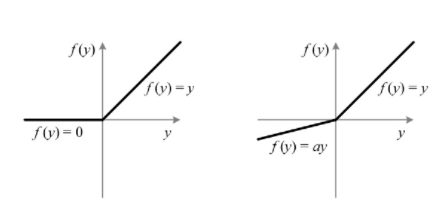允许不同通道的激活函数不一样。当时a=0，PReLU就变成了ReLU，而且 a 是一个可以学习的参数。
如果 a 是一个小的固定值， PReLU就变成了 LReLU （Leaky ReLU)。
LReLU目的是为了避免零梯度，实验表明LReLU相较 ReLU对准确率几乎没有提高，但是PReLU的参数 a 可以自适应学习，从而可以获得专门的激活函数
PReLU - 知乎
卷积核权重初始化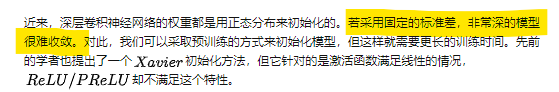展开全文神经网络 深度学习
• 深度学习——激活函数ReLu、LReLu、PReLu原理解析 简介 论文链接： https://arxiv.org/abs/1502.01852 由于项目需要，需要了解msra 权重初始化方法原理， 正好Kaiming 大神这篇论文提出了PReLu。本博客主要介绍...
深度学习——激活函数ReLu、LReLu、PReLu原理解析
简介
论文链接： https://arxiv.org/abs/1502.01852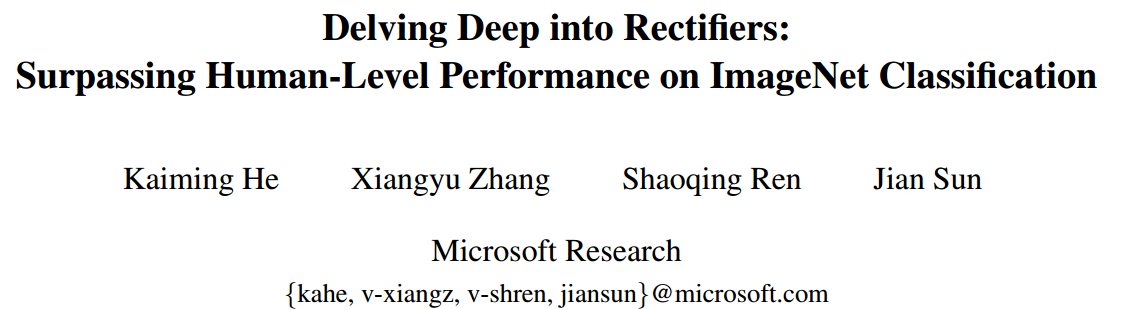由于项目需要，需要了解msra 权重初始化方法原理， 正好Kaiming 大神这篇论文提出了PReLu。本博客主要介绍PReLu。文中两个创新点是使用PReLu和msra初始化后，在I ImageNet 2012 classification dataset上，达到top5=4.94%,超越了人类识别水平。
原理分析
激活函数主要来做非线性变换，目前我知道激活函数有20多种，CV领域主要用到是sigmoid、ReLu系列。
ReLu公式

f

(

y

i

)

=

{

y

i

i

f

,

y

i

&gt;

0

0

i

f

,

y

i

≤

0

(

1

)

f(y_i)=\left\{ \begin{aligned} y_i &amp; &amp; {if, \quad y_i &gt;0 } \\ 0 &amp; &amp; {if ,\quad y_i \leq0 } \\ \end{aligned} \qquad(1)\right.

Leaky ReLu(LReLu)公式

f

(

y

i

)

=

{

y

i

i

f

,

y

i

&gt;

0

0.01

∗

y

i

i

f

,

y

i

≤

0

(

2

)

f(y_i)=\left\{ \begin{aligned} y_i &amp; &amp; {if, \quad y_i &gt;0 } \\ 0.01*y_i &amp; &amp; {if ,\quad y_i \leq0 } \\ \end{aligned} \qquad(2)\right.

PReLu公式

f

(

y

i

)

=

{

y

i

i

f

,

y

i

&gt;

0

a

i

∗

y

i

i

f

,

y

i

≤

0

(

3

)

f(y_i)=\left\{ \begin{aligned} y_i &amp; &amp; {if, \quad y_i &gt;0 } \\ a_i * y_i &amp; &amp; {if ,\quad y_i \leq0 } \\ \end{aligned} \qquad(3)\right.

The motivation of LReLU is to avoid zero gradients， PReLu是为了防止ReLu在小于0的情况下，梯度为0。根据文中作者介绍，LReLu相对与ReLu对模型影响相差不大。故作者提出了PReLu,通过学习

a

i

a_i

，让模型更加完美。其实

P

R

e

L

u

=

R

e

L

u

+

a

i

∗

m

i

n

(

0

,

y

i

)

PReLu= ReLu+a_i *min(0,y_i)

当

a

i

a_i

= 0.01时候，等于LReLu.

a

i

a_i

中的

i

i

是指

i

t

h

i^{th}

channel的参数，

y

i

y_i

是指

i

t

h

i^{th}

feature map.
PReLu 反向传播过程
来自上一层的delta为

δ

\delta

， 求

a

i

a_i

梯度 ，根据 chain rule当一个层中a_i每个通道不共享时候

∂

δ

∂

a

i

=

∑

i

=

1

w

∗

h

∂

δ

∂

f

(

y

i

)

∂

f

(

y

i

)

∂

a

i

=

∑

i

=

1

w

∗

h

∂

δ

∂

f

(

y

i

)

{

0

i

f

,

y

i

&gt;

0

y

i

i

f

,

y

i

≤

0

(

4

)

\frac{\partial \delta}{\partial a_i } = \sum_{i=1}^{w*h}\frac{\partial \delta}{\partial f(y_i)} \frac{\partial f(y_i)}{\partial a_i} = \sum_{i=1}^{w*h}\frac{\partial \delta}{\partial f(y_i)} \left\{ \begin{aligned} 0 &amp; &amp; {if, \quad y_i &gt;0 } \\ y_i &amp; &amp; {if ,\quad y_i \leq0 } \\ \end{aligned} \qquad(4)\right.

当一个层中只要一个a时，也就是所有通道共享。

∂

δ

∂

a

i

=

∑

j

=

1

c

h

a

n

n

e

l

∑

i

=

1

w

∗

h

∂

δ

∂

f

(

y

i

)

∂

f

(

y

i

)

∂

a

i

=

∑

j

=

1

c

h

a

n

n

e

l

∑

i

=

1

w

∗

h

∂

δ

∂

f

(

y

i

)

{

0

i

f

,

y

i

&gt;

0

y

i

i

f

,

y

i

≤

0

(

5

)

\frac{\partial \delta}{\partial a_i } = \sum_{j=1}^{channel}\sum_{i=1}^{w*h}\frac{\partial \delta}{\partial f(y_i)} \frac{\partial f(y_i)}{\partial a_i} = \sum_{j=1}^{channel}\sum_{i=1}^{w*h}\frac{\partial \delta}{\partial f(y_i)} \left\{ \begin{aligned} 0 &amp; &amp; {if, \quad y_i &gt;0 } \\ y_i &amp; &amp; {if ,\quad y_i \leq0 } \\ \end{aligned} \qquad(5)\right.

update

a

i

a_i

adopt the momentum method when updating ai

Δ

a

i

=

u

Δ

a

i

+

ε

∂

δ

∂

a

i

(

6

)

\varDelta a_i = u\varDelta a_i+\varepsilon \frac{\partial \delta}{\partial a_i}\qquad(6)

u

u

momentum，

ε

\varepsilon

代表学习速率。use ai = 0:25 as the initialization throughout this pape
实验结果
文中主要对

a

a

做了两种实验，一种是一个层只要一个a,也就是share模式。另外一种是一个层中每个通道都有一个a，wise模式。实验表明wise结果更好。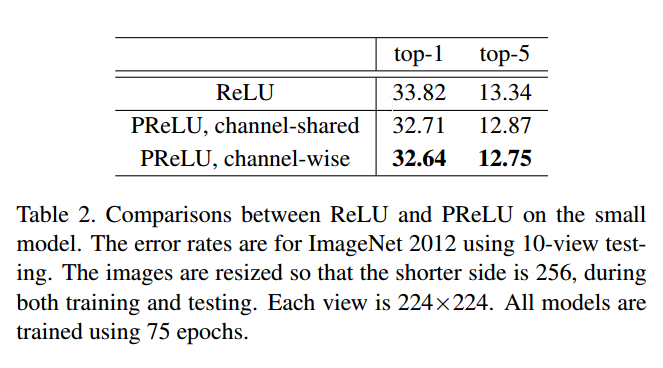展开全文• ReLU tensorflow中：tf.nn.relu(features, name=None)LReLU(Leaky-ReLU) 其中aiai是固定的。ii表示不同的通道对应不同的aiai. tensorflow中：tf.nn.leaky_relu(features, alpha=0.2, name=None)PReLU 其中aiai是...
ReLU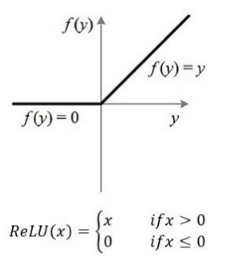tensorflow中：tf.nn.relu(features, name=None)
LReLU
(Leaky-ReLU)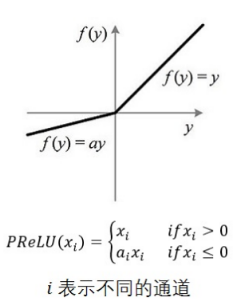其中aiai是固定的。ii表示不同的通道对应不同的aiai. tensorflow中：tf.nn.leaky_relu(features, alpha=0.2, name=None)
PReLU其中aiai是可以学习的的。如果ai=0ai=0，那么 PReLU 退化为ReLU；如果 aiai是一个很小的固定值（如ai=0.01ai=0.01），则 PReLU 退化为 Leaky ReLU（LReLU）。 PReLU 只增加了极少量的参数，也就意味着网络的计算量以及过拟合的危险性都只增加了一点点。特别的，当不同 channels 使用相同的aiai时，参数就更少了。BP 更新aiai时，采用的是带动量的更新方式（momentum）。 tensorflow中:没找到啊!
CReLU
（Concatenated Rectified Linear Units）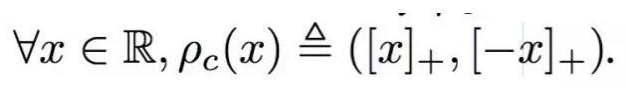tensorflow中：tf.nn.crelu(features, name=None)
ELU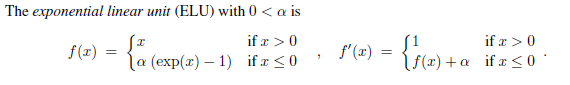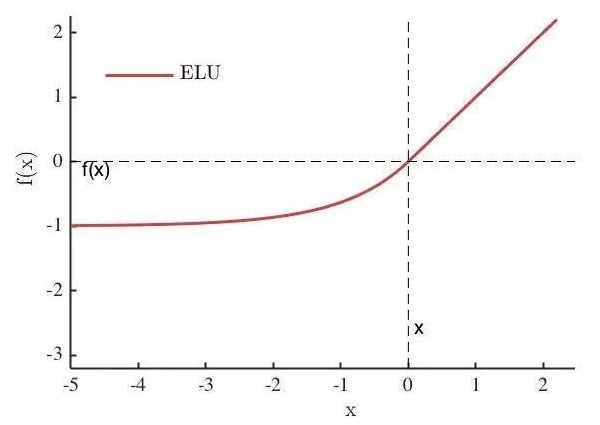其中α是一个可调整的参数，它控制着ELU负值部分在何时饱和。 右侧线性部分使得ELU能够缓解梯度消失，而左侧软饱能够让ELU对输入变化或噪声更鲁棒。ELU的输出均值接近于零，所以收敛速度更快 tensorflow中：tf.nn.elu(features, name=None)
SELU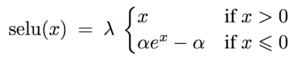经过该激活函数后使得样本分布自动归一化到0均值和单位方差(自归一化，保证训练过程中梯度不会爆炸或消失，效果比Batch Normalization 要好) 其实就是ELU乘了个lambda，关键在于这个lambda是大于1的。以前relu，prelu，elu这些激活函数，都是在负半轴坡度平缓，这样在activation的方差过大的时候可以让它减小，防止了梯度爆炸，但是正半轴坡度简单的设成了1。而selu的正半轴大于1，在方差过小的的时候可以让它增大，同时防止了梯度消失。这样激活函数就有一个不动点，网络深了以后每一层的输出都是均值为0方差为1。
tensorflow中：tf.nn.selu(features, name=None)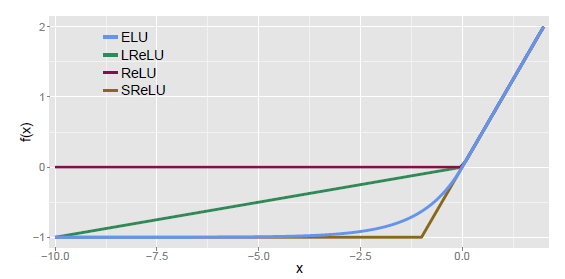展开全文深度学习 激活函数 ReLu PReLu
• # 绘制softmax函数 plt.plot(x,ReLU(x),c="blue",lw="2",label="ReLU") # 绘制ReLU函数 plt.plot(x,elu(x,0.1),c="darkviolet",lw="2",label="elu") # 绘制elu函数 plt.plot(x,LReLU(x),c="springgreen",lw="2",...

楼主最近在研究激活函数，索性将常用的激活函数进行了简单的整理，方便以后翻看，也希望能帮到你。

1、sigmoid函数

函数表达式：f(x) = 1/(1+e^-x)
函数特点：
优点：1.输出[0,1]之间；2.连续函数，方便求导。
缺点：1.容易产生梯度消失；2.输出不是以零为中心；3.大量运算时相当耗时（由于是幂函数）。

函数定义：
def sigmoid(x):
y = 1/(1+np.exp(-x))
return y


2、tanh函数

函数表达式：f(x) = (e^x-e^-x)/(e^x+e-x)
函数特点：
优点：1.输出[-1,1]之间；2.连续函数，方便求导；3.输出以零为中心。
缺点：1.容易产生梯度消失； 2.大量数据运算时相当耗时（由于是幂函数）。

函数定义：
def tanh(x):
y = (np.exp(x) - np.exp(-x))/(np.exp(x) + np.exp(-x))
return y


3、ReLu函数

函数表达式： f(x)=  当x<0  f(x)=0； 当x>=0 f(x)=x
函数特点：
优点：1.解决了正区间梯度消失问题；2.易于计算； 3.收敛速度快
缺点：1.输出不是以零为中心；2.某些神经元不能被激活，导致参数永远不能更新。

函数定义：
def ReLU(x):
y = []
for i in x:
if i >= 0:
y.append(i)
else:
y.append(0)
return y


4、elu函数

函数表达式： f(x)=  当x>=0  f(x)=x；  当x<0  f(x) =a(e^x -1)
函数特点：
优点：1.解决了正区间梯度消失问题；2.易于计算；3.收敛速度快；4.解决了某些神经元不能被激活问题；5.输出的均值为0
缺点：输出不是以零为中心

函数定义：
def elu(x, a):
y = []
for i in x:
if i >= 0:
y.append(i)
else:
y.append(a*(np.exp(i)-1))
return y


5、leaky ReLU函数

函数表达式： f(x)=  当x>=0  f(x)=x； 当x<0  f(x) =0.01x
函数特点：
优点：1.解决了正区间梯度消失问题；2.易于计算；3.收敛速度快；4.解决了某些神经元不能被激活
缺点：输出不是以零为中心

函数定义：
def LReLU(x):
y = []
for i in x:
if i >= 0:
y.append(i)
else:
y.append(0.01*i)
return y


6、softplus函数

函数表达式： y = ln(1+e^x)
函数定义：
def softplus(x):
y = np.log(1 + np.exp(x))
return y


7、softmax函数

函数表达式：输入信号的指数函数除以所有输入信号的指数和
函数特点：一般用在分类的输出层作为激活函数
优点：1.输出在[0,1]之间，可以当初概率。
缺点： 在实际问题中，由于幂运算需要时间，而且softmax不会影响各元素的大小，因此输出层的softmax激活函数一般被省略。
函数定义：
def softmax(x):
c = np.max(x)   # 解决溢出问题
exe_x = np.exp(x)
exe_s = np.sum(exe_x)
y = exe_x/exe_s
return y


绘制函数的代码

#!/usr/bin/env python
# -*- coding: utf-8 -*-
# Author：'ZhangML'
# Time:2021/1/2  15:56

from matplotlib import pyplot as plt
import numpy as np
import math

x = np.linspace(-6, 6, 200)
# 1.sigmoid函数的表达式：f(x) = 1/(1+e^-x)
# 函数特点：
# 优点：1.输出[0,1]之间；2.连续函数，方便求导。
# 缺点：1.容易产生梯度消失；2.输出不是以零为中心；3.大量运算时相当耗时（由于是幂函数）。

def sigmoid(x):
y = 1/(1+np.exp(-x))
return y

# 2.tanh的函数表达式： f(x) = (e^x-e^-x)/(e^x+e-x)
# 函数特点：
# 优点：1.输出[-1,1]之间；2.连续函数，方便求导；3.输出以零为中心。
# 缺点：1.容易产生梯度消失； 2.大量数据运算时相当耗时（由于是幂函数）。
def tanh(x):
y = (np.exp(x) - np.exp(-x))/(np.exp(x) + np.exp(-x))
return y

# 3. ReLu 的函数表达式： f(x)=  当x<0  f(x)0   当x>=0 f(x) = x
# 函数特点：
# 优点：1.解决了正区间梯度消失问题；2.易于计算； 3.收敛速度快
# 缺点：1.输出不是以零为中心；2.某些神经元不能被激活，导致参数永远不能更新。
def ReLU(x):
y = []
for i in x:
if i >= 0:
y.append(i)
else:
y.append(0)
return y
# return np.maximum(x,*100)   #可以利用np中的maximum方法表示，也可以用上述方法

# 4.elu 的函数表达式  f(x)   x>=0  f(x)=x   x<0  f(x) =a(e^x -1)
# 函数特点：
# 优点：1.解决了正区间梯度消失问题；2.易于计算；3.收敛速度快；4.解决了某些神经元不能被激活问题；5.输出的均值为0
# 缺点：输出不是以零为中心
def elu(x, a):
y = []
for i in x:
if i >= 0:
y.append(i)
else:
y.append(a*(np.exp(i)-1))
return y

# 5.leaky ReLU 的函数表达式  x>=0  f(x)=x   x<0  f(x) =0.01x
# 函数特点：
# 优点：1.解决了正区间梯度消失问题；2.易于计算；3.收敛速度快；4.解决了某些神经元不能被激活
# 缺点：输出不是以零为中心
def LReLU(x):
y = []
for i in x:
if i >= 0:
y.append(i)
else:
y.append(0.01*i)
return y

# 6.softplus函数的表达式： y = ln(1+e^x)
def softplus(x):
y = np.log(1 + np.exp(x))
return y

# softmax激活函数   softmax的表达式为：输入信号的指数函数除以所有输入信号的指数和
# 函数特点：一般用在分类的输出层作为激活函数
# 优点：1.输出在[0,1]之间，可以当初概率。
# 缺点： 在实际问题中，由于幂运算需要时间，而且softmax不会影响各元素的大小，因此输出层的softmax激活函数一般被省略。
def softmax(x):
c = np.max(x)   # 解决溢出问题
exe_x = np.exp(x)
exe_s = np.sum(exe_x)
y = exe_x/exe_s
return y

font1 = {'family': 'Times New Roman', 'weight': 'normal', 'size': 15}

# 绘制整张图
ax = plt.subplot(111)
plt.plot(x,sigmoid(x),c="lime",lw="2",label = "Sigmiod")      # 绘制sigmoid函数
plt.plot(x,tanh(x),c="deeppink",lw="2",label = "Tanh")        # 绘制tanh函数
plt.plot(x,softmax(x),c="green",lw="2",label = "Softmax")     # 绘制softmax函数
plt.plot(x,ReLU(x),c="blue",lw="2",label="ReLU")              # 绘制ReLU函数
plt.plot(x,elu(x,0.1),c="darkviolet",lw="2",label="elu")      # 绘制elu函数
plt.plot(x,LReLU(x),c="springgreen",lw="2",label="LReLU")     # 绘制LReLU函数
plt.plot(x,softplus(x),c="red",lw="2",label="Softplus")       # 绘制Softplus函数
plt.gca().spines["bottom"].set_position(("data",0))           # 将函数图像移动到x轴（0,0）
plt.gca().spines["left"].set_position(("data",0))             # 将函数图形移动到y轴（0,0）
plt.xticks(fontproperties='Times New Roman', fontsize=18, )
plt.yticks(fontproperties='Times New Roman', fontsize=18, )
ax.spines['right'].set_visible(False)
ax.spines['top'].set_visible(False)
plt.legend(loc="best", frameon=False, prop=font1)
plt.show()

运行效果如下图所示：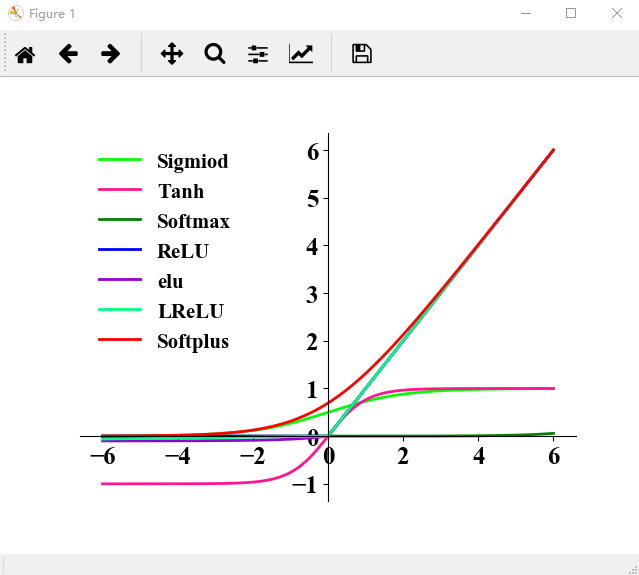这里有直接使用keras库中的激活函数绘图的，大同小异，想了解的可以看看，送上传送门，希望可以帮到你。
展开全文深度学习 python 神经网络
• 基于LReLU-Softplus激活函数的深度卷积神经网络.pdf
• LReLU公式： LReLU导数： LReLULReLU_derivatrive对比： 7.PReLU（隐层神经元输出） 对于 LReLU 中的α，通常都是通过先验知识人工赋值的。 然而可以观察到，损失函数对α的导数我们是可以求得的，因此可以将α...sigmod softmax Maxout Tanh ReLU
• ## ReLU、LReLU、PReLU、CReLU、ELU、SELU

万次阅读 多人点赞 2018-01-22 22:25:34
LReLU PReLU CReLU ELU SELUReLU tensorflow中：tf.nn.relu(features, name=None)LReLU(Leaky-ReLU) 其中aia_i是固定的。ii表示不同的通道对应不同的aia_i. tensorflow中：tf.nn.leaky_relu(features, alpha=...ReLU PReLU CReLU ELU
• Difference between ReLU、LReLU、PReLU、CReLU、ELU、SELU 激活函数 ReLU、LReLU、PReLU、CReLU、ELU、SELU 的定义和区别 ReLU tensorflow中：tf.nn.relu(features, name=None) RELU的优点即计算特别简单，...
• ReLULReLUPReLUCReLUELUSELUReLU tensorflow中：tf.nn.relu(features, name=None)LReLU(Leaky-ReLU) 其中aiai是固定的。ii表示不同的通道对应不同的aiai. tensorflow中：tf.nn.leaky_relu(features, alpha=0.2, ...
• l1 = self.lrelu(self.conv_first(x.view(-1, c, h, w))) feat_l1 = self.feature_extraction(feat_l1) # L2 feat_l2 = self.lrelu(self.conv_l2_1(feat_l1)) feat_l2 = self.lrelu(self.conv_l2_2(feat_l2)) # L3 ...深度学习 计算机视觉 算法 pytorch
• def lrelu(x, leak=0.2, name="lrelu"): return tf.maximum(x, leak*x) # 定义一个MLP全连接操作 def linear(input_, output_size, scope=None, stddev=0.02, bias_start=0.0, with_w=False): shape = input_....图像识别 人工智能
• lrelu(self.bn('d_bn1',output)) out.append(output1) # output1 = tf.contrib.keras.layers.GaussianNoise output = conv2d('d_con2', output1, 3, 64*2, stride=2, padding='SAME')#7*7 output2 = lrelu(self.bn('...算法 python 计算机视觉 tensorflow 神经网络
• activation_fn=lrelu def UNet(inputs, reg): # Unet conv1 = slim.conv2d(inputs, 32, [3, 3], rate=1, activation_fn=activation_fn, scope='g_conv1_1', weights_regularizer=reg) conv1 = slim.conv2d(conv1,...TensorFlow UNet U-Net
• with tf.name_scope("lrelu"): # adding these together creates the leak part and linear part # then cancels them out by subtracting/adding an absolute value term # leak: a*x/2 - a*abs(x)/2 # linear...
• LReLU、PReLU与RReLU 通常在LReLU和PReLU中，我们定义一个激活函数为 f(yi)={yi if (yi>0)aiyi if (yi≤0)f\left(y_{i}\right)=\left\{\begin{array}{ll} y_{i} & \text { if }\left(y_{i}>0\right) \\ a_{i} y_{i}...
• 今天小编就为大家分享一篇pytorch的batch normalize使用详解，具有很好的参考价值，希望对大家有所帮助。一起跟随小编过来看看吧
• Conv<a href="%105_convolutional_lrelu,%20%106_convolutional_conv_weights,%20%106_convolutional_conv_bias">auto_pad = 'SAME_LOWER', dilations = [1, 1], kernel_shape = [1, 1], ...
• mapping_nonlinearity = 'lrelu', # Activation function: 'relu', 'lrelu'. use_wscale = True, # Enable equalized learning rate? normalize_latents = True, # Normalize latent vectors (Z) before feeding ...GANS stylegan 图片生成 图片融合...